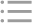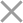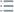Pour obtenir une clé
d'essai remplissez le formulaire ci-dessous
Team licenсe
Enterprise licence
** En cliquant sur ce bouton, vous acceptez notre politique de confidentialité.Demandez des tariffs
Nouvelle licence
Renouvellement de licence
--Sélectionnez la devise--
USD
EUR
RUB
* En cliquant sur ce bouton, vous acceptez notre politique de confidentialitéFree PVS-Studio license for Microsoft MVP specialists
** En cliquant sur ce bouton, vous acceptez notre politique de confidentialité.To get the licence for your open-source project, please fill out this form
** En cliquant sur ce bouton, vous acceptez notre politique de confidentialité.I am interested to try it on the platforms:
 Windows Linux macOS PVS-Studio for .NET Core JetBrains Rider
** En cliquant sur ce bouton, vous acceptez notre politique de confidentialité.Votre message a été envoyé.

Nous vous répondrons à

Si vous n'avez toujours pas reçu de réponse, vérifiez votre dossier
Spam/Junk et cliquez sur le bouton "Not Spam".
De cette façon, vous ne manquerez la réponse de notre équipe.

>
>
>
V3152. Potential division by zero. Vari…Introduction
Analyzing projects
On Windows
On Linux and macOS
Continuous use of the analyzer in software development
Deploying the analyzer in cloud Continuous Integration services
Managing analysis results
Additional configuration and resolving issues
Analyzer diagnostics
General Analysis (C++)
General Analysis (C#)
General Analysis (Java)
Diagnosis of micro-optimizations (C++)
Diagnosis of 64-bit errors (Viva64, C++)
Customer specific requests (C++)
MISRA errors
AUTOSAR errors
OWASP errors (C++)
OWASP errors (C#)
OWASP errors (Java)
Problems related to code analyzerContents

# V3152. Potential division by zero. Variable was compared to zero before it was used as a divisor. Check lines: N1, N2.

20 Fév 2020

The analyzer has detected a potential division-by-zero error.

A numeric variable is first compared with zero and then divided by without such a check. This means one of the two scenarios:

1) If the divisor variable has the value 0, an error will occur.

2) The division always yields a correct result because the divisor variable is never 0. In this case, the null check is unnecessary.

Consider the following example. Executing this code may result in throwing an exception:

``````int num = Foo();
if (num != 0)
variable1 = 3 / num;
variable2 = 5 / num;``````

If the value of 'num' happens to be zero, executing the '5 / num' expression will lead to an error. The analyzer reports this code by pointing at two lines: the first is where the division is executed and the second is where the divisor variable is checked for null.

Fixed code:

``````int num = Foo();
if (num != 0)
{
variable1 = 3 / num;
variable2 = 5 / num;
}``````

Consider another example. The division here is safe and the check is not needed:

``````List<string> list = CreateNonEmptyList();
var count = list.Count;
if (count == 0) {....}
var variable = 10 / count;``````

Suppose the 'CreateNonEmptyList' method always returns a non-empty list for 'list'. In that case, the code above will always work correctly and a division by zero will never occur.

Note: in this example, the analyzer does not produce the V3152 warning every time: if it can understand that the method always returns a non-empty list, it will instead issue a V3022 warning ("expression always true") on the check 'list.Count == 0'. If it fails to understand that (for example, because of a complicated sequence of reassignments or when the method is virtual, and so on), it will issue a V3152 warning. The type of the warning to be issued will depend on the implementation of the 'CreateNonEmptyList' method.

To eliminate the warning, remove the check 'if (list == null || list.Count == 0)'. In this case, it has no practical use and can only confuse the maintainer.

Fixed code:

``````List<string> list = CreateNonEmptyList();
var variable = 10 / list.Count;``````

Another case where this warning is issued is when the null check and the division operation occur in different branches of if-else or switch statements, for example:

``````if (lines.Count == 1)
{
if (num != 0)
variable = 10 / num;
}
else
{
variable = 10 / num;
}``````

In this example, even though execution can never follow both branches at one run and will instead follow only one of them, the fact that the variable is compared with zero in one of the branches is a sign that it can have the value 0 in the other branch. If that happens, a division by zero will occur when the other branch gets control.

Fixed version:

``````if (lines.Count == 1)
{
if (num != 0)
variable = 10 / num;
}
else
{
if (num != 0)
variable = 10 / num;
}``````

As an alternative to removing the check to eliminate a false positive, you can also use a warning-suppression comment, for example:

``variable = 10 / num; //-V3152``

This diagnostic is classified as:Nous utilisons des cookies pour améliorer votre expérience de navigation. En savoir plus